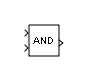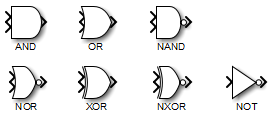Main Content

# Logical Operator

Perform specified logical operation on input

• Library:
• Simulink / Commonly Used Blocks

Simulink / Logic and Bit Operations

HDL Coder / Commonly Used Blocks

HDL Coder / Logic and Bit Operations

•## Description

The Logical Operator block performs the specified logical operation on its inputs. An input value is true (`1`) if it is nonzero and false (`0`) if it is zero.

You select the Boolean operation connecting the inputs with the Operator parameter list. If you select `rectangular` as the Icon shape property, the name of the selected operator displays on the block icon. If you select `distinctive` as the Icon shape property, the name of the selected operator does not display on the block icon. This table shows supported operations:

OperationDescription

AND

TRUE if all inputs are TRUE

OR

TRUE if at least one input is TRUE

NAND

TRUE if at least one input is FALSE

NOR

TRUE when no inputs are TRUE

XOR

TRUE if an odd number of inputs are TRUE

NXOR

TRUE if an even number of inputs are TRUE

NOT

TRUE if the input is FALSE

If you select `distinctive` as the Icon shape, the block appearance indicates its function. Simulink® software displays a distinctive shape for the selected operator, conforming to the IEEE® Standard Graphic Symbols for Logic Functions.To specify the number of input ports, use the Number of input ports parameter. The output type is specified using the Output data type parameter. An output value is `1` if TRUE and `0` if FALSE.

Note

The output data type should represent zero exactly. Data types that satisfy this condition include signed and unsigned integers, and any floating-point data type.

The size of the output depends on input vector size and the selected operator:

• If the block has more than one input, any nonscalar inputs must have the same dimensions. For example, if any input is a 2-by-2 array, all other nonscalar inputs must also be 2-by-2 arrays.

Scalar inputs are expanded to have the same dimensions as the nonscalar inputs.

If the block has more than one input, the output has the same dimensions as the inputs (after scalar expansion) and each output element is the result of applying the specified logical operation to the corresponding input elements. For example, if the specified operation is AND and the inputs are 2-by-2 arrays, the output is a 2-by-2 array whose top left element is the result of applying AND to the top left elements of the inputs, and so on.

• For a single vector input, the block applies the operation (except the NOT operator) to all elements of the vector. The output is always a scalar.

• The NOT operator accepts only one input, which can be a scalar or a vector. If the input is a vector, the output is a vector of the same size containing the logical complements of the input vector elements.

When configured as a multi-input XOR gate, this block performs an addition modulo two operation as mandated by the IEEE Standard for Logic Elements.

## Ports

### Input

expand all

First input signal, specified as a scalar, vector, or matrix.

Data Types: `half` | `single` | `double` | `int8` | `int16` | `int32` | `int64` | `uint8` | `uint16` | `uint32` | `uint64` | `Boolean` | `fixed point`

Second input signal, specified as a scalar, vector, or matrix.

Data Types: `half` | `single` | `double` | `int8` | `int16` | `int32` | `int64` | `uint8` | `uint16` | `uint32` | `uint64` | `Boolean` | `fixed point`

Nth input signal, specified as a scalar, vector, or matrix.

#### Dependencies

To enable additional input ports, use the Number of input ports parameter.

Data Types: `half` | `single` | `double` | `int8` | `int16` | `int32` | `int64` | `uint8` | `uint16` | `uint32` | `uint64` | `Boolean` | `fixed point`

### Output

expand all

Output signal, consisting of zeros and ones, with the same dimensions as the input. You control the output data type with the Require all inputs and output to have the same data type and Output data type parameters.

Data Types: `half` | `single` | `double` | `int8` | `int16` | `int32` | `int64` | `uint8` | `uint16` | `uint32` | `uint64` | `Boolean` | `fixed point`

## Parameters

expand all

### Main

Select the logical operator to apply to block inputs.

• `AND` — T if all inputs are TRUE

• `OR` — TRUE if at least one input is TRUE

• `NAND` — TRUE if at least one input is FALSE

• `NOR` — TRUE when no inputs are TRUE

• `XOR` — TRUE if an odd number of inputs are TRUE

• `NXOR` — TRUE if an even number of inputs are TRUE

• `NOT` — TRUE if the input is FALSE

#### Programmatic Use

 Block Parameter: `Operator` Type: character vector Values: ```'AND' | 'OR' | 'NAND' | 'NOR' | 'XOR' | 'NXOR' | 'NOT'``` Default: `'AND'`

Specify the number of block inputs as a positive integer.

#### Programmatic Use

 Block Parameter: `Inputs` Type: character vector Values: positive integer Default: `'2'`

#### Dependencies

This parameter is not available when you set the Operator to `NOT`.

Specify the shape of the block icon.

• `rectangular` — Results in a rectangular block that displays the name of the selected operator.

• `distinctive` — Use the graphic symbol for the selected operator as specified by the IEEE standard.

#### Programmatic Use

 Block Parameter: `IconShape` Type: character vector Values: `'rectangular' | 'distinctive'` Default: `'rectangular'`

Specify the sample time as a value other than -1. For more information, see Specify Sample Time.

#### Dependencies

This parameter is not visible unless it is explicitly set to a value other than `-1`. To learn more, see Blocks for Which Sample Time Is Not Recommended.

#### Programmatic Use

 Block Parameter: `SampleTime` Type: character vector Values: scalar or vector Default: `'-1'`

### Data Type

To require that all block inputs and the output have the same data type, select this check box. When you clear this check box, the inputs and output can have different data types.

#### Programmatic Use

 Block Parameter: `AllPortsSameDT` Type: character vector Values: `'off' | 'on` Default: `'off'`

Specify the output data type. When you select:

• `boolean` — The block output has data type `boolean`.

• ```Inherit: Logical (see Configuration Parameters: Optimization)``` — The block uses the Implement logic signals as Boolean data configuration parameter to specify the output data type (see Implement logic signals as Boolean data (vs. double)) .

Note

This option supports models created before the `boolean` option was available. Use one of the other options, preferably `boolean`, for new models.

• `fixdt(1,16)` — The block output has the specified fixed-point data type `fixdt(1,16)`.

Tip

Click the buttonto display the Data Type Assistant, which helps you set the data type attributes. For more information, see Specify Data Types Using Data Type Assistant.

• `<data type expression>` — The block output has the data type you specify as a data type expression, for example, `Simulink.NumericType`.

Tip

To enter a built-in data type (`double`, `single`, `int8`, `uint8`, `int16`, `uint16`, `int32`, or `uint32`), enclose the expression in single quotes. For example, enter `'double'` instead of `double`.

#### Programmatic Use

 Block Parameter: `OutDataTypeStr` Type: character vector Values: ```'Inherit: Logical (see Configuration Parameters: Optimization)' | 'boolean' | 'fixdt(1,16)' | ''``` Default: `'boolean'`

## Block Characteristics

 Data Types `Boolean` | `double` | `fixed point` | `half` | `integer` | `single` Direct Feedthrough `yes` Multidimensional Signals `yes` Variable-Size Signals `yes` Zero-Crossing Detection `no`

## See Also

Introduced before R2006a

## Support

#### Model-Based Design for Embedded Control Systems

Download white paper